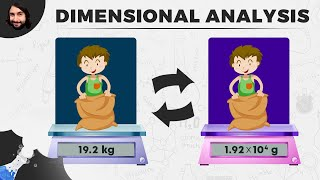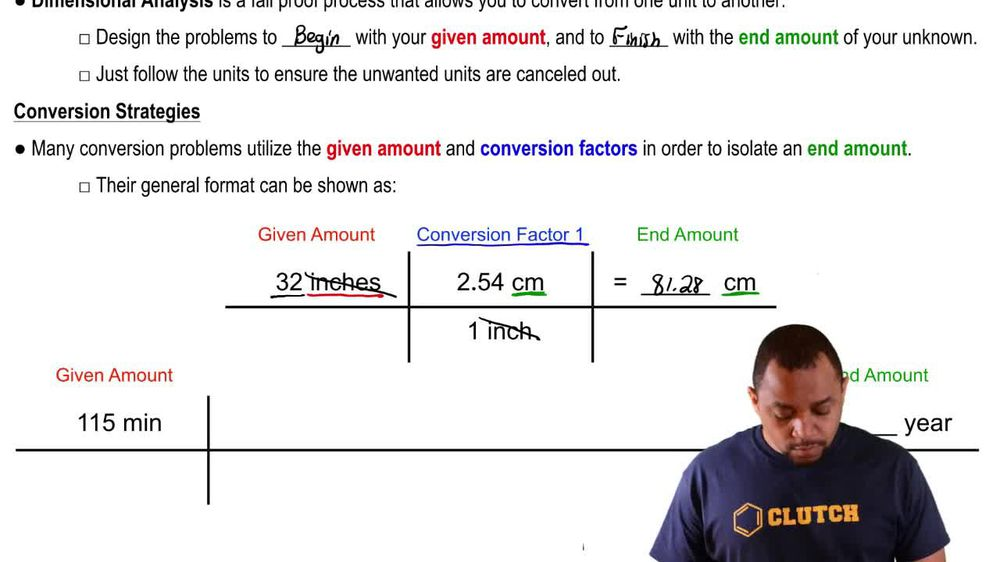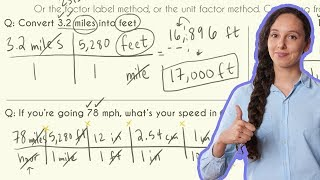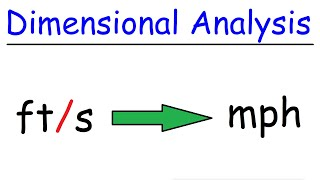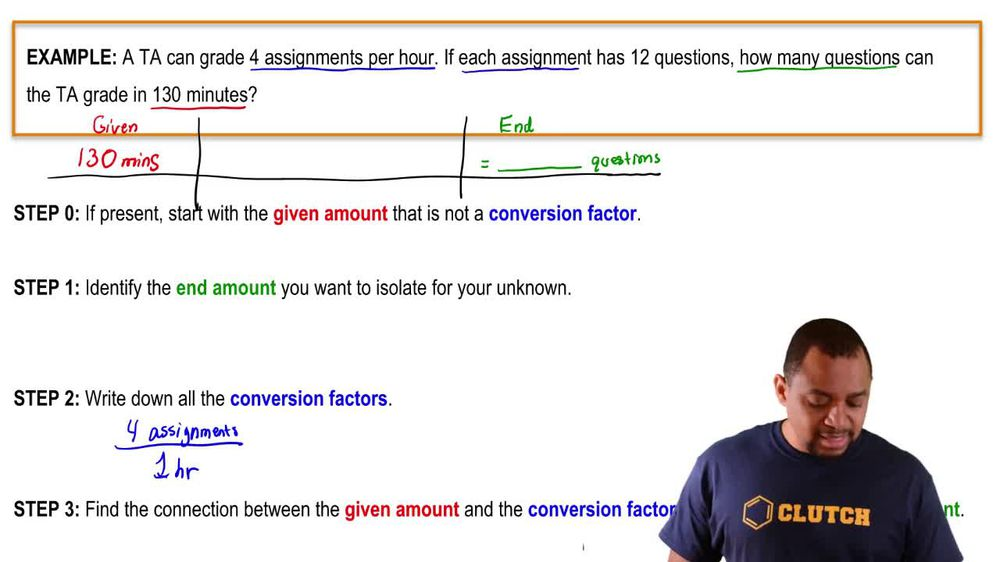Start typing, then use the up and down arrows to select an option from the list.
1. 1. Intro to General Chemistry2. Dimensional Analysis
Problem

# The world record for the women’s outdoor 20,000-meter run, set in 2000 by Tegla Loroupe, is 1:05:26.6 (seconds are given to the nearest tenth). What was her average speed, expressed in miles per hour with the correct number of significant figures? (Assume that the race distance is accurate to 5 significant figures)

Relevant Solution3m
Play a video:
everyone today. We have a question asked us to calculate the speed and MPH for the following freestyle swimming, world records, 100 m freestyle, 46.1 seconds, 100 yard freestyle, 44.8 seconds And we need to consider three significant figures for 100 m and 100 yards in the calculation. So first we're gonna start out with our 100 m and for this one are important. Conversion is 0. mi equals one kilometer. So now we can put that into an equation. So we're gonna start with our 100 m Over 46.1 seconds. We're going to multiply that by one km Over 10 to the 3rd m And we're going to multiply that by 0.6-1, four miles over one kilometer. And we're going to multiply that by our 60 seconds over one minute And multiply that by 60 minutes over one hour. And here our meters are canceling out our kilometers are canceling out, our miles are canceled, Our miles are not canceling out. Our miles are staying, our seconds are canceling out and our minutes are canceling out giving us MPH. So that equals for 0. MPH. And now, for our second calculation are important, conversion is one mile Equals 1760 yards. So we're gonna start with our 100 yards Per 44.8 seconds. And we're going to multiply that by our one mile Over 1760 yards And we're going to multiply that by 60 seconds over one minute And multiply that by 60 minutes over one hour. So our yards are going to cancel out, and our seconds are going to cancel out, and our minutes are going to cancel out, leaving us with MPH, And that equals 4.57 mph. And those are our final answers. Thank you for watching. Bye.